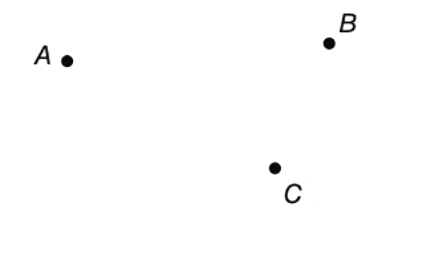Chapter P.3, Problem 5EElementary Geometry For College St...

7th Edition
Alexander + 2 others
ISBN: 9781337614085

Solutions

Chapter
SectionElementary Geometry For College St...

7th Edition
Alexander + 2 others
ISBN: 9781337614085
Textbook Problem

How many lines can be drawn that contain both points A and B ?How many lines can be drawn that contain points A B and C ?To determine

To find:

The number of lines that can be drawn which contain both points A and B and also A, B and C.

Explanation

Calculation:

Given,

Two points can be joined by a line.

Thus, only one line can be drawn which contain both the points A and B.

Non-collinear points cannot be joined by a single line

Still sussing out bartleby?

Check out a sample textbook solution.

See a sample solution

The Solution to Your Study Problems

Bartleby provides explanations to thousands of textbook problems written by our experts, many with advanced degrees!

Get Started

CHECKPOINT CHECKPOINT 3. Find the average value of .

Mathematical Applications for the Management, Life, and Social Sciences

A population of N = 7 scores has a mean of = 13. What is the value of X for this population?

Essentials of Statistics for The Behavioral Sciences (MindTap Course List)

Calculate y'. 24. y=1/x+x3

Single Variable Calculus: Early Transcendentals, Volume I

True or False: is a convergent series.

Study Guide for Stewart's Single Variable Calculus: Early Transcendentals, 8th